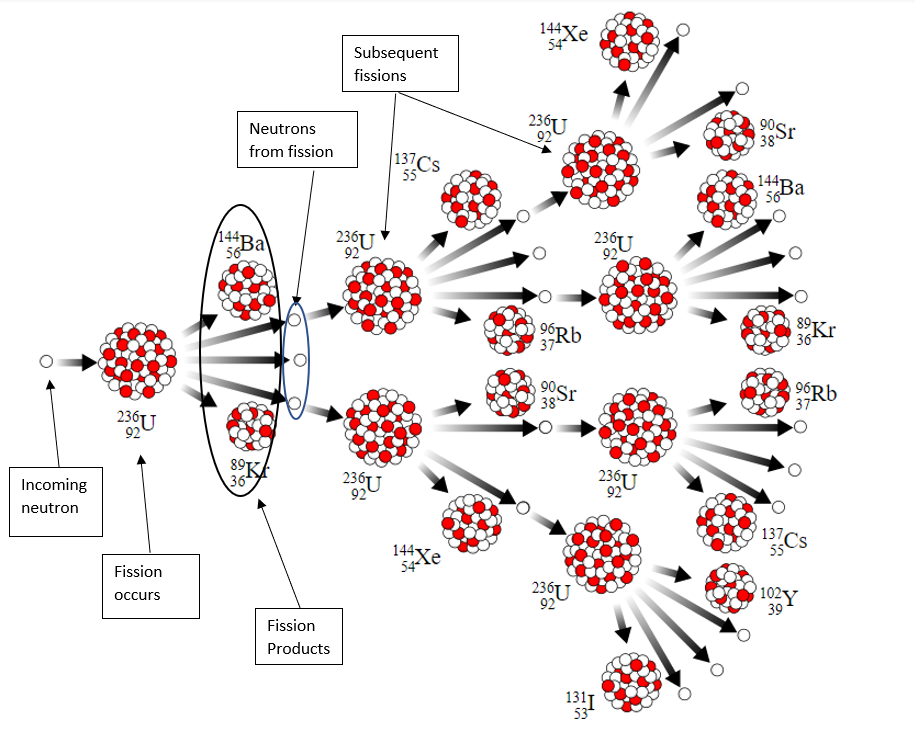# Nuclear Fission

A nuclear fission reaction is the splitting of an atom when bumbarded with neutrons (following absoprtion). The fission can occur from a fast or thermal (slow) neutron and can also occur spontaneously (particularly in very heavy elements). The atom splits into two smaller atoms called fission fragments and ejects neutrons which can cause subsequent fissions. This is called a chain reaction.A fissile nuclide can fission from a neutron of any energy. A Fissionable nuclide can fission but only from neutrons which sufficient energy above a threshold. Examples are given below. Notice that fissile nuclides are generally have an odd value of atomic mass because the binding energy is larger for pairs of nucleons. A fertile nuclide can be converted to a fissile material through nuclear reactions/decay. This process is referred to as breeding.

 Fissile $$^{235}U, \; ^{233}U, \; ^{239}Pu, \; ^{241}Pu$$ Fissionable $$^{238}U, \; ^{240}Pu$$ Fertile $$^{232}Th, \; ^{234}U, \; ^{238}U$$

On average, 2-3 neutrons are released form fission. This quantity is denoted $$\nu$$ and depends on the fissioning nuclide and incoming neutron energy. When a neutron is absorbed, it is either captured, followed by decay, or causes fissioning. The capture-to-fission ratio is given by $$\alpha = \frac{\sigma_\gamma}{\sigma_a}$$ where $$\sigma_i$$ is a cross section representing the probability of the different interactions. The reproduction factor is the number of neutrons emitted per absorption and is given by $$\eta = \frac{\nu}{1+\alpha}$$.

Nuclear fission reactions are critical when one nuetron produced from fission goes on to induce another fission. This reaction is stable. The criticality is a measure of how close the reactor is to a self-sustaining, critical reaction. It is given by:

$$k_{eff} = \frac{\text{rate of neutron production}}{\text{rate of neutron loss by absorption and leakage}}$$

A reactor can be critical, subcritical (eventually shuts down) or supercritical (rate of power increases drastically). The Reactivity is closely related to criticality and is defined as:

$$\rho = 1-\frac{1}{k_{eff}}$$

The units of reactivity are $$[mk]$$ or one part in one thousand. Although small, nuclear reactions occur very quickly, thus dangerous power levels can occur for small supercritical reactivities.

 Subcritical $$k_{eff} < 1$$ $$\rho < 0$$ Critical $$k_{eff} = 1$$ $$\rho = 0$$ Supercritical $$k_{eff} > 1$$ $$\rho > 0$$

A reactor is operating at $$k_{eff} = 0.99965$$. What is the reactivity? Is the reaction critical?